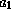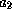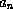Common Lisp the Language, 2nd EditionNext: Array Access Up: Arrays Previous: Arrays

# 17.1. Array Creation

Do not be daunted by the many options of the function make-array. All that is required to construct an array is a list of the dimensions; most of the options are for relatively esoteric applications.

[Function]
make-array dimensions &key :element-type :initial-element :initial-contents :adjustable :fill-pointer :displaced-to :displaced-index-offset

This is the primitive function for making arrays. The dimensions argument should be a list of non-negative integers that are to be the dimensions of the array; the length of the list will be the dimensionality of the array. Each dimension must be smaller than array-dimension-limit, and the product of all the dimensions must be smaller than array-total-size-limit. Note that if dimensions is nil, then a zero-dimensional array is created. For convenience when making a one-dimensional array, the single dimension may be provided as an integer rather than as a list of one integer.

An implementation of Common Lisp may impose a limit on the rank of an array, but this limit may not be smaller than 7. Therefore, any Common Lisp program may assume the use of arrays of rank 7 or less. The implementation-dependent limit on array rank is reflected in array-rank-limit.

The keyword arguments for make-array are as follows:

:element-type
This argument should be the name of the type of the elements of the array; an array is constructed of the most specialized type that can nevertheless accommodate elements of the given type. The type t specifies a general array, one whose elements may be any Lisp object; this is the default type.X3J13 voted in January 1989 (ARRAY-TYPE-ELEMENT-TYPE-SEMANTICS)   to change typep and subtypep so that the specialized array type specifier means the same thing for discrimination purposes as for declaration purposes: it encompasses those arrays that can result by specifying element-type as the element type to the function make-array. Therefore we may say that if type is the :element-type argument, then the result will be an array of type (array type); put another way, for any type A,

```(typep (make-array ... :element-type 'A ...)
'(array A)))
```:initial-element
This argument may be used to initialize each element of the array. The value must be of the type specified by the :element-type argument. If the :initial-element option is omitted, the initial values of the array elements are undefined (unless the :initial-contents or :displaced-to option is used). The :initial-element option may not be used with the :initial-contents or :displaced-to option.

:initial-contents
This argument may be used to initialize the contents of the array. The value is a nested structure of sequences. If the array is zero-dimensional, then the value specifies the single element. Otherwise, the value must be a sequence whose length is equal to the first dimension; each element must be a nested structure for an array whose dimensions are the remaining dimensions, and so on. For example:

```(make-array '(4 2 3)
:initial-contents
'(((a b c) (1 2 3))
((d e f) (3 1 2))
((g h i) (2 3 1))
((j k l) (0 0 0))))
```

The numbers of levels in the structure must equal the rank of the array. Each leaf of the nested structure must be of the type specified by the :type option. If the :initial-contents option is omitted, the initial values of the array elements are undefined (unless the :initial-element or :displaced-to option is used). The :initial-contents option may not be used with the :initial-element or :displaced-to option.

This argument, if specified and not nil, indicates that it must be possible to alter the array's size dynamically after it is created. This argument defaults to nil.X3J13 voted in June 1989 (ADJUST-ARRAY-NOT-ADJUSTABLE)   to clarify that if this argument is non-nil then the predicate adjustable-array-p will necessarily be true when applied to the resulting array; but if this argument is nil (or omitted) then the resulting array may or may not be adjustable, depending on the implementation, and therefore adjustable-array-p may be correspondingly true or false of the resulting array. Common Lisp provides no portable way to create a non-adjustable array, that is, an array for which adjustable-array-p is guaranteed to be false.:fill-pointer
This argument specifies that the array should have a fill pointer. If this option is specified and not nil, the array must be one-dimensional. The value is used to initialize the fill pointer for the array. If the value t is specified, the length of the array is used; otherwise the value must be an integer between 0 (inclusive) and the length of the array (inclusive). This argument defaults to nil.

:displaced-to
This argument, if specified and not nil, specifies that the array will be a displaced array. The argument must then be an array; make-array will create an indirect or shared array that shares its contents with the specified array. In this case the :displaced-index-offset option may be useful. It is an error if the array given as the :displaced-to argument does not have the same :element-type as the array being created. The :displaced-to option may not be used with the :initial-element or :initial-contents option. This argument defaults to nil.

:displaced-index-offset
This argument may be used only in conjunction with the displaced-to option. It must be a non-negative integer (it defaults to zero); it is made to be the index-offset of the created shared array.

When an array A is given as the :displaced-to argument to make-array when creating array B, then array B is said to be displaced to array A. Now the total number of elements in an array, called the total size of the array, is calculated as the product of all the dimensions (see array-total-size). It is required that the total size of A be no smaller than the sum of the total size of B plus the offset n specified by the :displaced-index-offset argument. The effect of displacing is that array B does not have any elements of its own but instead maps accesses to itself into accesses to array A. The mapping treats both arrays as if they were one-dimensional by taking the elements in row-major order, and then maps an access to element k of array B to an access to element k+n of array A.

If make-array is called with each of the :adjustable, :fill-pointer, and :displaced-to arguments either unspecified or nil, then the resulting array is guaranteed to be a simple array (see section 2.5).X3J13 voted in June 1989 (ADJUST-ARRAY-NOT-ADJUSTABLE)   to clarify that if one or more of the :adjustable, :fill-pointer, and :displaced-to arguments is true, then whether the resulting array is simple is unspecified.Here are some examples of the use of make-array:

```;;; Create a one-dimensional array of five elements.
(make-array 5)

;;; Create a two-dimensional array, 3 by 4, with four-bit elements.
(make-array '(3 4) :element-type '(mod 16))

;;; Create an array of single-floats.
(make-array 5 :element-type 'single-float))

;;; Making a shared array.
(setq a (make-array '(4 3)))
(setq b (make-array 8 :displaced-to a
:displaced-index-offset 2))
;;; Now it is the case that:
(aref b 0) == (aref a 0 2)
(aref b 1) == (aref a 1 0)
(aref b 2) == (aref a 1 1)
(aref b 3) == (aref a 1 2)
(aref b 4) == (aref a 2 0)
(aref b 5) == (aref a 2 1)
(aref b 6) == (aref a 2 2)
(aref b 7) == (aref a 3 0)
```

The last example depends on the fact that arrays are, in effect, stored in row-major order for purposes of sharing. Put another way, the indices for the elements of an array are ordered lexicographically.

Compatibility note: Both Lisp Machine Lisp, as described in reference , and Fortran [15,3] store arrays in column-major order.

[Constant]
array-rank-limit

The value of array-rank-limit is a positive integer that is the upper exclusive bound on the rank of an array. This bound depends on the implementation but will not be smaller than 8; therefore every Common Lisp implementation supports arrays whose rank is between 0 and 7 (inclusive). (Implementors are encouraged to make this limit as large as practicable without sacrificing performance.)

[Constant]
array-dimension-limit

The value of array-dimension-limit is a positive integer that is the upper exclusive bound on each individual dimension of an array. This bound depends on the implementation but will not be smaller than 1024. (Implementors are encouraged to make this limit as large as practicable without sacrificing performance.)X3J13 voted in January 1989 (FIXNUM-NON-PORTABLE)   to specify that the value of array-dimension-limit must be of type fixnum. This in turn implies that all valid array indices will be fixnums.[Constant]
array-total-size-limit

The value of array-total-size-limit is a positive integer that is the upper exclusive bound on the total number of elements in an array. This bound depends on the implementation but will not be smaller than 1024. (Implementors are encouraged to make this limit as large as practicable without sacrificing performance.)

The actual limit on array size imposed by the implementation may vary according to the :element-type of the array; in this case the value of array-total-size-limit will be the smallest of these individual limits.

[Function]
vector &rest objects

The function vector is a convenient means for creating a simple general vector with specified initial contents. It is analogous to the function list.

```(vector...)
== (make-array (list n) :element-type t
:initial-contents (list...))
```Next: Array Access Up: Arrays Previous: Arrays

[email protected]